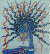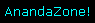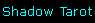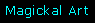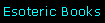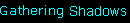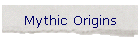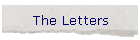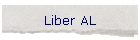© linda falorio, 1978, 1996, 1998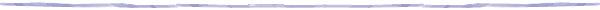##### III.73: "Paste the sheets from right to left and from top to bottom: then behold!"

Applying the instructions from III.73 to II.75, 76 ~ i.e. reading the numbers from right to left ~ and taking 3XY as a mathematical expression, when we apply the E.Q. values to the text: 98 + 42 + 198 + 4 + 2 + 8 + 3 + 6 + 4 = 365, the number of days in the year. This is just the sort of correspondence to be expected if our work is to remain in harmony with the ancient traditions of the Ollaves in constructing alphabets based on seasonal cycles and cosmological mathematics. Listed for 365 we find HEKT THE FROG-HEADED GODDESS AND LADY OF TRANSFORMATION; WITHIN THE INNERMOST SANCTUARIES OF THE ADEPT'S NON-BEING; AT THE BORDERLAND OF SLEEP AND WAKING ARE THE LIERS IN WAIT; THE NUMBER 5 DENOTES THE EARTH AS FLESH CONGEALED FROM BLOOD.

II.75,76, numbers and words included, added right to left in the above manner yields 506, which is also the sum of  8 + 80 + 418 ~ i.e. the list of numbers from I.46. 506 is 5 united with 6, symbolic of uniting the Microcosm with the Macrocosm ~ the Great Work accomplished ~ where find THE ENTRANCE TO THE UNDERWORLD IS IN THE CIRCULAR KIVAS.

RPSTOVAL adds to 76, the number of the verse in which the word appears, where we find listed in our Book of Numbers, THE GREAT GATE, THE TOWER OF MAAT, WINGED MOUTH, BLACK ISIS, THE DARK GOD, THE HURRICANE, MIGHTY ANGEL, ANDROGYNE, ADVERSARY, OPPOSITE IMAGE, RAPID LIGHT, STOPS TIME, REVERSES.

AL, the Arabic word for God has traditionally been used as a suffix to designate qualities of godhead. Returning to II.75-76, if we consider AL as a suffix rather than as an integral part of the sentence, the total numeration of the letters is 143, the same as the numeration of the numbers, and of the phrase AYE! LISTEN TO THE WORDS. For 143 we also list THE MOTHER OF ALL LIVING; THE NOCTURNAL LILITH; MAAT THE DOUBLE-WANDED ONE; THE GODDESS SIRIUS; THE ORACULAR SERPENT; THE DARK GOD OF THIS KALA; THE VIBRATION OF THIS KALA; GATE OF THE EVENING STAR.

Adding the suffix AL, the total numeration of the sentence II. 75-76 adds to 301, Hebrew ASh ~ 'fire". 301 is 43, the STAR, multiplied by 7: i.e. it is the seven-fold Star of Babalon, the sigil of Liber AL.

Another way to "paste the sheets from right to left and from top to bottom" would be via the use of a magic square of 8 by 8, which is Hadit's sacred number. 8 x 8 = 64; 1/64 = .0156 ~ 156, the number of the "Whore", Babalon. Along these same curious lines, the cube root of 31, the "Key" to Liber AL according to Achad's reckoning, equals the elusive pi, exact to three decimal places.

When we paste the sheets from right to left and from top to bottom in an 8 by 8 square using the letters and number equivalents from the cryptic sentence II.75.76, we find that each of the thirty figures is used once, and we are left with a 3 by 3 subsidiary square, which we may fill by going back to the beginning of the sentence, using the first six letters: RPSTOV, and deleting AL, the god-termination. RPSTOV equals 61, and in this magick square, or Kamea, the sum of the ranks, files, and diagonals equals 610, which is 61 x 10 ~ the expansion of Nothing into material existence.

In the subsidiary Kamea using the letters RPSTOV, the sum of the ranks and files each equals 88 ~ the sacred number of Hadit multiplied by the number of Magick.

The total sum of ranks, files, and diagonals equals 253 ~ the Maatian 23 multiplied by the number of Magick. 253 x 2 = 506 = 22 x 23: which is the manifestation of the double current of HruMaKhu. Curiously, 253/5 = 50.6.

Listed for 253 we find IN THE SCARLET WOMAN IS ALL POWER GIVEN; THE VOID IN WHICH OUR POTENTIAL IS CONCEALED; THE BLACK PLANET WHERE THERE ARE 2 SUNS; OXOSSI THE BLIND HUNTER IN STARLIGHT.

Another interesting Kamea of this sentence uses the phrase ALGMOR in a 3 by 3 magick square. The sum of the ranks plus files for this square equals that most interesting number ~ 156.KAMEA OF "LIBER AL vel LEGIS"

 L A V O T S P R C V O E X Y I P R O M G L A Y S O E K B A L X T F I M O B G E O S P R M K M O V O T P I E O V A V O S F R O C L
 S P R O T P V O S
 G L A O M L R O G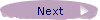© AnandaZone 1998 - 2004 All contents © Linda Falorio unless otherwise noted. The English Qabalah™, EQ™, and E.Q.™ are trademarks of Anandazone anandazone@anandazone.nu Linda Falorio / Fred Fowler Pittsburgh, PA 15224 U S A